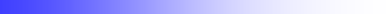A.Kaminsky® SUBJECTIVE  PHYSICS Unfortunately, the English version of our site is much less developed than the Russian version. Nevertheless, you will be able to use the automatic translation function of the pages that interests you. Here we offer you an article which contains the basic ideas of our approach. "The fundamental process of nature lies outside space–time but generates events that can be located in space–time." H.Stapp   Anatomy of quantum superposition (3- bit Universe) We are studying the simplest model of a finite deterministic world. Here, we have attempted to answer the following question: what our artificial world would look like from the point of view of an observer (subject), placed in our modeled finite world? To do this, it's necessary to formulate abstract model of the observer. Only in that way is it possible to answer this complicated question. Herein, we have endeavored to show the quantum-similar character of the laws, discovering by the subjective observer.  As a consequence of this exercise, we can now assume that  physical laws of our real world have a similar origin.1. Introduction The central point of the theory, developed in this article, will be postulated, based on pseudo-classical determinism on the basic level of matter organization.  This point of view leads us to the conception of the world as a finite automaton with a large number of states. See for example . We introduce the fundamental states, as states of an algorithm, or states of a finite automaton. However, we should take into account that the observer will occupy part of the computational resources (cells) of the world automaton. Consequently, he will not have access to each fundamental state of the world, taken separately, because some of them constitute its own knowledge. Therefore, nature would appear to him to be much rougher and granular than it really is. Such an observer will be referred to as the subjective observer (SO). The hypothetical observer, which can survey our world as a whole, will be referred to as the objective observer (OO). The question of OO’s existence is beyond the bounds of physics so we will apply to the point of view of OO only in an abstract sense for greater clarity. The error of traditional scientific method lays in attempts to explain the phenomena of nature from the point of view of an objective observer.   Further on we will show that a quantum mechanical description of the world is the description of the subjective observer. Quantum states are not just mere mathematical devices which enable physicists to make statistical predictions. Quantum states are a single reality for the subjective observer. This modeling situation is similar to Gödel’s Incompleteness . How would real world dynamics appear to an observer who is a part of the world?  It's a very difficult problem and we can not provide a comprehensive answer to it.  This being said, we can construct a simple model and test its behavior. Our approach validates the idea of hidden variables and may yield clues to the foundation of quantum mechanics. In the past, hidden variable theories were dismissed by physicists for a number of reasons: The first reason leads to von-Neumann’s theorem and experimental demonstrations of the violation of Bell's inequalities. The second reason is that the majority of researchers have no need for such theories. Although compelling, the aforementioned arguments do not serve to justify a lack of investigation into hidden variable theory.2. Physical incompleteness and hidden time   Let us consider a simple deterministic system, consisting of a set of N=2n states. Where n is the length of a word of the world's state. The current time in this system is equivalent to the count of the current algorithmic step. Such time is called an algorithmic time. World dynamics can be described by means of a trajectory on an n-dimensional binary cube. Because the algorithmic trajectory is not self-crossing, it presents the discrete analogue of an ergodic trajectory. In addition, it presents a Hamilton cycle on the binary cube. The number of n-bits Universes is equal to the number of Hamilton cycles on the n-dimensional binary cube. If n=3, then the number of possible Universes is (23)!=40320.   Let us assume further that m from n bits of our model Universe are occupied by the observer. And they are necessary for description of his internal states. Such a model we will call an (n, m)- model . The space of fundamental states can therefore be represented as a tensor product of the observer's space and the space of the rest of the world.   W=H Ä R      (1) Here H- is known as Hilbert space in quantum mechanics and R – is a space of hidden states.  Due to a type of physical incompleteness and the limitations of our nature (m